Electricity & Megnetism

# Electric generator

Electric generator is a device that can convert different forms of energy , such as mechanical, chemical and solar energy, into electrical energy. The most common operating principle among generators is electromagnetic induction . This process occurs when a set of conductive turns, arranged inside the generators, are placed between magnets , so that the rotation of these coils can produce a large amount of electric current, used to feed the most varied types of electric circuits .

## Introduction to electric generators

Electric generators are made to produce electrical energy from some other energy source. In the case of batteries , for example, they transform the energy contained in chemical bonds into electric current, whereas the reactors used in hydroelectric or thermoelectric plants are used dynamos, which work according to the Faraday-Lenz law .

Electric generators can be classified as ideal or real generators . In practice, however, no generator is ideal, that is, there are no 100% efficient generators, in all of them, some of the energy produced will be dissipated by the action of friction forces and the Joule effect .

Real generators are those that have a non-zero internal resistance, these devices produce a potential difference that is called electromotive force ( EMF) – which is not a force , but an electrical voltage , measured in volts, however. the name was kept for historical reasons. When connected to electrical circuits, real generators cannot establish a potential difference equal to their EMF, the voltage produced by them is slightly lower in these cases and is called useful or usable voltage.

The difference between the voltage that the generator is capable of producing and the voltage that it is capable of delivering is the energy that it itself dissipates in the form of heat , vibrations and sounds during its operation. The measure of this lost energy is called the dissipated voltage.

The symbol used to denote ideal generators in electrical circuits is shown in the following figure. Watch:The symbol used for real generators is shown below, note that in this type of generator there is an indication of an internal resistance: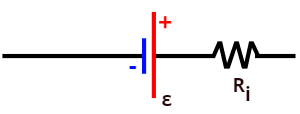According to the conventional direction of electric current , generators that are connected to electrical circuits must be traveled from the terminal with the lowest electrical potential (negative sign) towards the terminal with the highest electrical potential (positive sign). When this happens, the charge carriers of the electric current will be gaining energy as they pass through the generator.

## Energy balance in the generator – characteristic equation

The relationship between useful, total and dissipated voltages is described by the characteristic equation of generators. It is a 1st degree equation , in which the voltage is written as a function of the electric current. Look at this formula: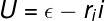U – useful voltage (V)

ε – electromotive force (V)

i – generator internal resistance (Ω)

i – electric current (A)

## Characteristic curve of generators

The characteristic curve of generators is a graph that relates the useful voltage to the electric current . It is a descending line, as shown below: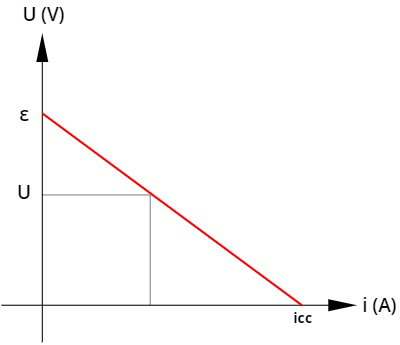dc – short circuit current

The graph shows two particular measures ε and i cc. The electromotive force (ε) measures the maximum electrical potential that the generator is capable of producing. This value, however, is only obtained when the generator is disconnected from the circuit, so that no energy is dissipated by the passage of electric current.

The short-circuit current , in turn, measures the maximum electrical current that can be produced by the generator, therefore, it is necessary that the generator terminals are directly connected by a conductor wire. This type of connection is known as a short circuit , since the electrical current does not pass through the elements of the electrical circuit.

The characteristic curve of generators is particularly useful when you want to find out what the internal resistance of a generator is, in which case it is enough that the electromotive force (ε) is divided by the short-circuit current, as shown in the following formula: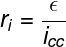## Generator power

The power of a generator is a measure of how much energy it is able to convert into electrical energy each second. The power quantity is defined by the ratio between energy, in joules, and time, in seconds, therefore, it is measured in joules per second , also known as watts (W).

As with the electrical potential, generators have a total power, a useful power and a dissipated power.  To obtain them, we simply multiply both sides of the characteristic equation of generators by the electric current i.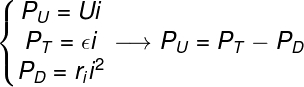T – total power (W)

U – useful power (W)

D – dissipated power (W)

## Generator Yield

Generator efficiency is a dimensionless quantity used to determine how efficient a generator is in providing electrical energy . Efficiency is calculated by dividing the useful voltage by the electromotive force or by dividing the useful power by the total power. Check out: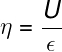η – generator efficiency

After dividing the quantities mentioned above, it is possible to multiply the result obtained by 100 to express the generator’s efficiency as a percentage .

## Exercises solved

Question 1) A generator of electromotive force equal to 12.0 V and internal resistance of 0.5 Ω is crossed by an electric current of 4.0 A. The approximate efficiency of this generator, for this electric current intensity is:

a) 57%

b) 83%

c) 62%

d) 75%

e) 93%

Resolution

alternative b. Before calculating the efficiency of this generator, it is necessary to find out what its useful voltage is, for that, we will use the characteristic equation of generators, see how: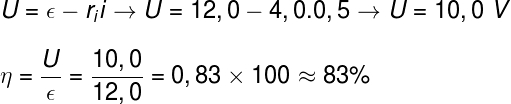Once the useful voltage of the generator has been determined, it is enough to divide it by the electromotive force and multiply the result by 100, doing this, we find an efficiency of approximately 83%.

Question 2) A battery has an electromotive force of 1.7 V, however, it establishes a voltage of 1.5 V when connected to a circuit. Knowing that the internal resistance of this cell is 0.25 Ω, calculate the power dissipated by the resistance of this cell.

a) 0.16 W

b) 0.5 W

c) 6.0 W

d) 12.0 W

e) 8.0 W

Template : Letter A

Resolution

alternative a. To calculate the dissipated power, we need to know both the resistance of the battery and the current that passes through it, so, through the characteristic equation of generators, we will find the value of the electric current: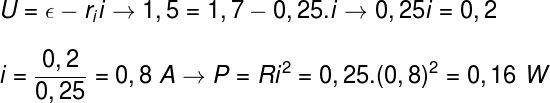After having calculated the electric current, it was enough to use the formula for the dissipated power (P = Ri²), in this way, we obtained a dissipated power of 0.16 W.